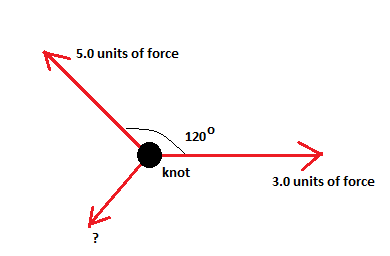# The figure shows three ropes tied together in a knot. One of your friends pulls on a rope with...

## Question:

The figure shows three ropes tied together in a knot. One of your friends pulls on a rope with 3.0 units of force and another pulls on a second rope with 5.0 units of force. What force (magnitude and direction) must you apply to the third rope to keep the knot from moving?Consider two vectors {eq}\displaystyle {\vec{a}} {/eq} and {eq}\displaystyle {\vec{b}} {/eq} making an angle {eq}\displaystyle {\theta} {/eq} with respect to each other. Then their resultant vector has a magnitude {eq}\displaystyle {\sqrt{a^2+b^2+2ab \cos \theta}} {/eq}. The resultant makes an angle {eq}\displaystyle {\alpha} {/eq} with the vector {eq}\displaystyle {\vec{a}} {/eq} given by, {eq}\displaystyle { \tan \alpha=\frac{b \sin \theta}{a+b \cos \theta}} {/eq}.

Here it is given that the three ropes are tied to form a know with two of your friends pulling it with forces of magnitudes say {eq}\displaystyle {a} {/eq} and {eq}\displaystyle {b} {/eq}, with {eq}\displaystyle {a=3} {/eq} and {eq}\displaystyle {b=5} {/eq} at an angle of {eq}\displaystyle {\theta=120^{\circ}} {/eq} with each other.

Clearly the resultant force has a magnitude,

{eq}\displaystyle { \sqrt{3^2+5^2+2\times 3\times 5 \cos 120}}=\sqrt{19} {/eq}.

The angle made by this resultant force with the horizontal vector {eq}\displaystyle {\vec{a}} {/eq} is,

{eq}\displaystyle { \tan \alpha=\frac{5 \sin 120}{3+5 \cos 120}}=8.66 {/eq}

This gives,

{eq}\displaystyle {\alpha=83.41^{\circ}} {/eq}.

Therefore to keep the knot in place you need to pull the third rope with a force of {eq}\displaystyle {\sqrt{19}\ N} {/eq} in the exactly opposite direction. This direction would make an angle of {eq}\displaystyle {180-83.41=96.59^{\circ}} {/eq} clockwise with the 3 N force.